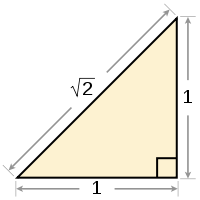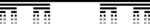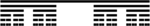# Here be (mathematical) dragons

by Rishidev Chaudhuri

Monsters lurk in the artificial paradises that mathematicians construct for themselves, swimming, slouching and fluttering into view from the corners of the imagination. These strange beasts – odd constructions or unexpected consequences of axioms – trouble the mathematical mind and threaten to expel the mathematician into chaos. By embodying the limits and the peculiarities of the concepts we use they force us to clarify, to draw distinctions where none had been drawn before, and to interrogate which objects we may meaningfully speak of and which properties we can assume. They also serve as a fascinating and wondrous gallery of the oddities of the mathematical universe, reflecting elegant and treacherous subtleties in their construction. Many mathematicians collect menageries of counterexamples and unusual cases, much as a naturalist might display upon returning from some distant continent.A number of these emerge from the delicate structure of the number line, and the ideas of infinity and continuity that follow. One of the first and best-known is the square root of 2, along with the (probably apocryphal) story of the trouble it caused the Pythagoreans. Unlike most of the numbers we normally encounter, the square root of 2 cannot be written as a fraction (the ratio of two integers – what a mathematician would call a rational number)1. Allegedly the Pythagoreans, driven by their number mysticism and belief in a cosmos governed by whole numbers and their ratios, tried to keep this discovery a secret, going so far as to execute a member for revealing it to the outside world.

In fact, the situation is much worse. Not just most, but effectively all of the real numbers (the numbers on the number line; the numbers that can be written with possibly infinite decimal expansions) cannot be written as fractions. The numbers that we deal with everyday, numbers like ½, ¼, 5 and so on, are an infinitely small proportion of the total, lost in a host of numbers that we never dream of. This realization is the entry into the strangeness that is the real number line, and is a glimpse at the long attempt to grapple with and understand infinity and continuity.

We run into complications as soon as we try to quantify the relative sizes of the rational and irrational numbers (irrational meaning “cannot be written as a fraction”). We can't just count the number of elements of an infinite set, then count the elements of another and compare them, at least not in the usual sense. Instead, we abstract away from what we usually do when we count things. To say that a set has 4 elements is to say that each element of the set can be paired up with the numbers {1, 2, 3, 4}, with nothing left over on either side. So the set {John, George, Paul, Ringo} can be matched like so: John->1, George->2, Paul->3, Ringo->4 and we can say that the set has 4 elements. This approach can be extended to the infinite. If we can pair a set to the set {1, 2, 3, 4, 5, ….}, where the …. indicates that we continue for all natural numbers, then the set is called countably infinite or just countable. One of the odd properties of infinite sets (and one of the reasons that they are more complicated to count), is that parts of the set can have the same size as the whole (in much the same way that subtracting 1 from an infinite number shouldn't change its size). So the set {2, 4, 6, 8, ….}, with the dots now indicating all even numbers, is the same size as {1, 2, 3, 4, ….} because we can match the elements up: 2->1, 4->2, 6->3 and so on. Every element from each set will be paired with an element from the other, and nothing will be left over. In fact, everything that we can write as an infinite list can be paired up with the natural numbers this way: pair the first element with 1, the second element with 2 and so on. The set of rational numbers can be listed out in an infinite list that leaves no rational number out, so the number of rational numbers is the same as the number of integers. Extrapolating from these examples suggests that all infinite sets are of the same size, and this is what people thought for a long time.

But the real numbers cannot be listed out this way, no matter how small a segment of the real line we look at, and no matter how ingenious we are with our listing. It can be proved that no matter how we try to set up the list, we will always leave numbers out2. Indeed we will leave out basically every real number. So there are different orders of infinity, and the number of real numbers is infinitely greater than the number of integers (which is also the number of rationals). The rational numbers occupy an infinitely small fraction of the number line or, put another way, if we could pick a number from the number line truly at random, the chance of it being rational is 0. Since the real numbers are made up of the rational numbers and the irrationals, this means that effectively all of the real numbers are irrational.

When Cantor first proposed this in 1874 it was very controversial, leading to attacks from other mathematicians and from religious figures (who, among other things, argued that positing multiple levels of infinity was a challenge to the infinity of God). Even today, certain schools of thought believe that this vision is an inappropriate extension of our finite concepts to things that we cannot meaningfully speak of.

Much as in life, it is common in mathematics to find that behavior that initially seemed pathological and disruptive to the conceived order of things is actually the norm. The objects that we are familiar with turn out to be isolated examples on the edges of turbulent seas of strangeness. And so, even irrational numbers as simple as the square root of 2 are negligible. In fact, the set of irrational numbers that can be written by adding, subtracting, multiplying, dividing and taking the roots of rational numbers a finite number of times is itself a vanishingly small set, no bigger than the integers.

Despite making up effectively none of the number line, in another sense the rationals are ubiquitous. A rational number lies between any two numbers, no matter how close they are. In fact, infinitely many rational numbers lie between any two numbers. And none of the other numbers on the number line can be separated from the rationals. By this I mean that if we were to draw a small circle around a particular number, this circle would contain infinitely many rational numbers no matter how small the circle. So the rational numbers can be used to describe an irrational number to any degree of precision, just not exactly. This is what we do when, for example, we find the square root of 2 on a computer or write pi as 3.141592. If we group the rationals together with all the points that cannot be separated from them by a small enough circle (what a mathematician would call taking the closure of the set of rationals), we get back the whole number line. The rationals are an infinitesimal skeleton on which the number line lies.

All of these properties of the number line are the same as for a segment of the line. So the piece between 0 and 1 has as many rational numbers and as many real numbers as the whole number line, and they are organized in the same way.The Cantor set is an odd and beautiful construction that throws more light on these ideas. To construct the Cantor set, we start by taking the piece of the number line between 0 and 1 and removing the middle third, leaving two pieces. Now we have two lines, so we do the same thing to each, removing their middle thirds. And then we repeat that with the remaining pieces on and on, on each step removing the middle thirds of all the remaining line segments. The resulting structure is self-similar (a fractal); if we zoom in on any of the sub-pieces, it looks like the original.

It now turns out that we've removed the entire length of the line, and the set we're left with is just a collection of disconnected points, like the finest imaginable dust scattered on the line. We started with a line of length 1 (between 0 and 1) and removed a line of length 1/3 on the first round. We removed two lines of length 1/9 on the second, four lines of length 1/27 on the third, and so on. If we add together the total length of all these lines we get 1. So the total length removed is what we started with and the resulting set has zero length, just like a point.

On the other hand we're left with the same number of points we started with: the same as the number of real numbers and infinitely greater than the number of rationals. We can pair every real number on the intact line between 0 and 1 with a different point in the Cantor set with nothing left over. This is much more surprising than showing that the set of even integers is the same size as the set of integers. Here, we've got rid of what we might think of as one of the defining features of the real numbers – the set we're left with has length 0. So we've separated out two features of the continuous number line that we might have thought had to go together – the infinitely greater number of points than the rationals, and the property of non-zero length (which a finite or countable collection of points won't have). So, in some sense the existence of the Cantor set tells us that we can take the points on the real line and arrange them so that they have zero length.

Now how are these points distributed? Well, there are large parts of the line where there are none of them (all of the segments we've removed, in fact and, as we just saw, these add up to the entire length of the line). On the other hand, if you pick a particular point in the Cantor set and look at the region within a small distance away, you'll find infinitely many of the points in the set. So, for example, if you look at 1/3, and draw a circle of size 0.00001 around it, you'll find infinitely many of the points in the set. All of them will lie to the left, in this case. But, unlike with the rationals, the only points that can't be separated from the members of the Cantor set are the members of the Cantor set themselves. So while there are infinitely many more members of the Cantor set, they are entirely self-contained.The Cantor set leads quite naturally to several other unusual and intricate constructions. If, instead of removing a constant fraction from each remaining line (i.e. removing a third from the first line, removing a third from each of the two remaining lines and so on), we remove a decreasing fraction from each new line, we get a fat Cantor set. This set has the same number of points as the Cantor set, and they are disconnected in the same way, but now they have length greater than 0. So we can get nonzero length without having connected segments of the number line in our set.The so-called Devil's staircase is built on the number line between 0 and 1 and is flat on any of the intervals removed during the construction of the Cantor set. So the staircase is flat on 100% of the length of the line. And yet its values on the points of the Cantor set are such that it not only increases from a value of 0 to 1, it does so in a continuous way, which means that it never jumps or misses a value.

There are many more unsettling constructions like the Cantor set and the Devil's staircase and this is a fascinating subject to explore. Wikipedia is generally excellent for math, and is a great place to start exploring if you're interested in finding out more.

********************

1The proof is quite simple and elegant, and is certainly worth looking at if you haven't seen it before: http://en.wikipedia.org/wiki/Square_root_of_2#Proof_by_infinite_descent

2This can be seen from Cantor's famous diagonalization: http://en.wikipedia.org/wiki/Cantor%27s_diagonal_argument

All the images have been taken from Wikipedia